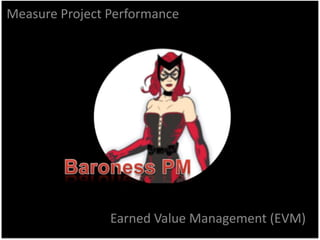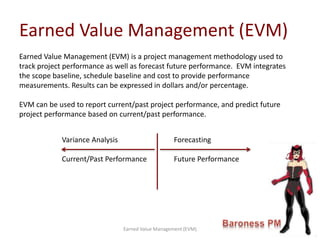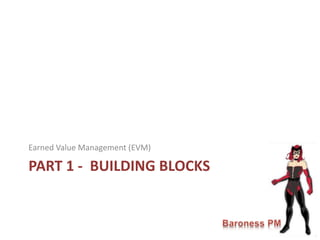Se está descargando tu SlideShare. ×

# Track Project Performance - Earned Value Management

Anuncio
Anuncio
Anuncio
Anuncio
Anuncio
Anuncio
Anuncio
Anuncio
Anuncio
Anuncio
AnuncioCargando en…3
×

1 de 20 Anuncio

# Track Project Performance - Earned Value Management

Earned Value Management (EVM) is a project management methodology used to track project performance as well as forecast future performance. This presentation will walk you through the calculations for EVM as well as performance reporting

Earned Value Management (EVM) is a project management methodology used to track project performance as well as forecast future performance. This presentation will walk you through the calculations for EVM as well as performance reporting

Anuncio
Anuncio

### Track Project Performance - Earned Value Management

1. 1. Earned Value Management (EVM) Measure Project Performance
2. 2. Earned Value Management (EVM) Earned Value Management (EVM) Earned Value Management (EVM) is a project management methodology used to track project performance as well as forecast future performance. EVM integrates the scope baseline, schedule baseline and cost to provide performance measurements. Results can be expressed in dollars and/or percentage. EVM can be used to report current/past project performance, and predict future project performance based on current/past performance. Variance Analysis Forecasting Current/Past Performance Future Performance
3. 3. PART 1 - BUILDING BLOCKS Earned Value Management (EVM)
4. 4. Earned Value Management (EVM) Starts with 3 building blocks that will be used in all EVM calculations. Budgeted amount planned to be spent at a point in time of the project Planned Value (PV) Earned Value (EV) Actual Cost (AC) Measurement of completed work according to the budget at a point in time of the project lifecycle Cost incurred for the work performed at a point in time of the project lifecycle Earned Value Management (EVM)
5. 5. Earned Value Management (EVM) Scenario: You are assigned to manage a 12 month project estimated to cost \$36,000 at the end of the project. Today is the end of month 3 and you are 20% complete. According to the Finance Department you have spent \$7,500 to date. Planned Value (PV) 1. Calculate % Planned. 3 months / 12 months = .25 2. Calculate \$ Planned. \$36,000 * .25 = \$9,000 Planned Value (PV) Earned Value (EV) Actual Cost (AC) Earned Value (EV) 1. Determine % Complete = .20 2. Calculate \$ Earned = 36,000 * .20 = 7,000 Actual Cost (AC) 1. Actual cost provided = \$7,500 Earned Value Management (EVM)
6. 6. PART 2 - VARIANCE ANALYSIS Earned Value Management (EVM)
7. 7. Earned Value Management (EVM) Variance Analysis can be used to monitor project performance based on schedule and cost. Each method can be expressed in a dollar value or percentage. Schedule Variance • Schedule Variance (SV) Project schedule performance expressed in dollars • Schedule Performance Index (SPI) Project schedule performance expressed in a percentage Cost Variance • Cost Variance (CV) Project cost performance expressed in dollars • Cost Performance Index (CPI) Project cost performance expressed in a percentage Earned Value Management (EVM)
8. 8. • Schedule Variance (SV) –Project performance expressed in dollars. SV = EV – PV SV = 7,000 – 9,000 SV = (2,000) • Schedule Performance Index (SPI) – Project performance expressed as a percent. SPI = EV / PV SPI = 7,000 / 9,000 SPI = .78 Earned Value Management (EVM) Schedule Variance Analysis uses EV and PV to calculate a variance to the project schedule. Earned Value Management (EVM) Earned Value Management Planned Value PV \$9,000 Earned Value EV \$7,000 Actual Cost AC \$7,500
9. 9. • Cost Variance (CV) –Project performance expressed in dollars. CV = EV – AC CV = 7,000 – 7,500 CV = (500) • Cost Performance Index (CPI) – Project performance expressed as a percent. CPI = EV / AC CPI = 7,000 / 7,500 CPI = .93 Earned Value Management (EVM) Cost Variance Analysis uses EV and AC to calculate a variance to the project budget (cost). Earned Value Management Planned Value PV \$9,000 Earned Value EV \$7,000 Actual Cost AC \$7,500 Earned Value Management (EVM)
10. 10. PART 3 - FORECASTING Earned Value Management (EVM) Earned Value Management (EVM)
11. 11. Earned Value Management (EVM) We can also use EVM to forecast future project spending. Estimate at Completion (EAC) can be calculated using the 3 following methods: Project will continue to perform at the budgeted rate EAC = AC + (BAC – EV) Budgeted Rate Current CPI Current CPI & SPI Project will continue to perform at the current CPI: EAC = BAC / CPI Project will continue to perform at the current CPI & SPI: EAC = AC + [(BAC – EV) / (CPI * SPI)] Earned Value Management (EVM) Estimate at Completion (EAC)
12. 12. Estimate at Completion (EAC) – Budgeted Rate: EAC = AC + (BAC – EV) EAC = 7,500 + (36,000 – 7,000) EAC = 7,500 + 29,000 EAC = \$36,500 Earned Value Management (EVM) Forecasting using Estimate at Completion (EAC) at the Budgeted rate, assumes the project will continue to perform at the currently budgeted rate. Earned Value Management (EVM)
13. 13. Estimate at Completion (EAC) – Current CPI EAC = BAC / CPI EAC = 36,000 / .78 EAC = \$46,153.84 Earned Value Management (EVM) Forecasting using Estimate at Completion at the current CPI, assumes the project will continue to perform at the Cost Performance Index (CPI) Earned Value Management (EVM)
14. 14. Estimate at Completion (EAC) – Current CPI & SPI EAC = AC + [(BAC – EV) / (CPI * SPI)] EAC = 7,500 + [(36,000 – 7,000) / (.78 * .93)] EAC = 7,500 + [29,000 / .7254] EAC = 7,500 + 39,977.94 EAC = 47,477.94 Earned Value Management (EVM) Forecasting using Estimate at Completion at the current CPI & SPI, assumes the project will continue to perform at the Cost Performance Index (CPI) and Schedule Performance Index (SPI) Earned Value Management (EVM)
15. 15. To Complete Performance Index (TCPI) Based on Balance at Completion: TCPI = [(BAC – EV) / (BAC - AC)] TCPI = [(36,000 – 7,000) / (36,000 – 7,500)] TCPI = (29,000) / (28,500) TCPI = 1.01 Earned Value Management (EVM) Earned Value Management (EVM) To Complete Performance Index (TCPI) Measure of schedule performance on a project.
16. 16. To Complete Performance Index (TCPI) Based on Estimate to Complete: TCPI = (BAC - EV) / (EAC – AC) TCPI = (36,000 – 7000) / (36,500 – 7,500) TCPI = 29,000 / 29,000 TCPI = 1.0 Earned Value Management (EVM) Earned Value Management (EVM) To Complete Performance Index (TCPI) Measure of schedule performance on a project.
17. 17. PART 4 - PERFORMANCE REVIEWS Earned Value Management (EVM)
18. 18. JAN FEB MAR APR MAY JUN JUL AUG SEP OCT NOV DEC PV 3,000 6,000 9,000 12,000 15,000 18,000 21,000 24,000 27,000 30,000 33,000 36,000 EV 3,240 5,400 7,200 10,000 13,000 15,000 AC 3,500 7,000 10,000 14,000 17,500 21,000 0 5,000 10,000 15,000 20,000 25,000 30,000 35,000 40,000 Earned Value Management (EVM) Performance Reviews Earned Value Management (EVM) Planned Value PV Actual Cost AC Earned Value EV Time Cost BAC EACForecastingVariance Analysis Status Date Variance Analysis: We can graph the project performance to show the project is currently behind schedule and over budget Forecast: We can also graph the estimated future performance to show the project will exceed the budget.
19. 19. APPENDIX – EVM QUICK REFERENCE Earned Value Management (EVM)
20. 20. Earned Value Management (EVM) Earned Value Management – Quick Reference Name Description Equation Planned Value PV Budgeted amount planned to be spent Budgeted Rate * % Planned Earned Value EV Measurement of completed work according to the budget % Complete * BAC Actual Cost AC Cost incurred for the work performed Budget at Completion BAC Total Planned Value for the project Cost Variance CV The difference in budgeted amount and actual spend at a given time. CV = EV - PV Schedule Variance SV The difference in Planned value and earned value indicating if the project is ahead or behind schedule SV = EV - PV Variance at Completion VAC The difference between the planned budget at completion and estimate to complete VAC = BAC - EAC Cost Performance Index CPI Measurement of Cost efficiency expressed as a percentage CPI = EV / PV Schedule Performance Index SPI Measurement of schedule efficiency expressed as a percentage SPI = EV / PV Estimate at Completion EAC Estimated cost to complete the project Based on current CPI Based on budgeted rate Based on current CPI & SPI EAC = BAC/CPI EAC = AC + (BAC – EV) EAC = AC + [(BAC – EV) / (CPI * SPI)] Estimate to Complete ETC Expected cost to complete work remaining ETC = EAC - AC To Complete Performance Index TCPI Measurement of performance required to meet the project goals. Expressed as a percentage. EAC = AC + [(BAC – EV) / (CPI * SPI)] TCPI = [(BAC – EV) / (BAC - AC)]